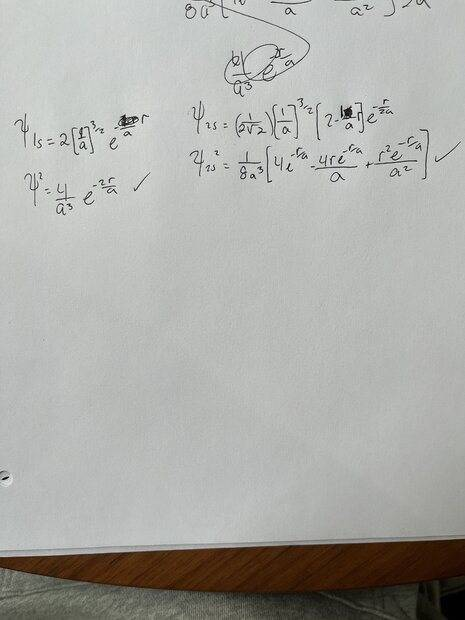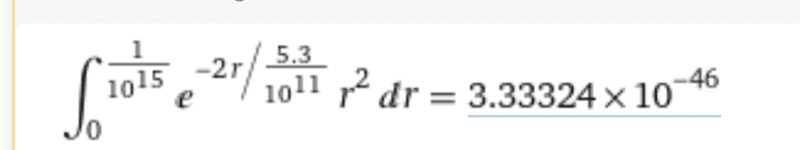# Probability of electron in hydrogen nucleus for 1s and 2s wave-functions

guyvsdcsniper
Homework Statement:
For the 1s and 2s wave functions, find the probability of the electron to be found inside the nucleus (a sphere of radius R). A typical nucleus has a radius of 1e-15 m.
Relevant Equations:
Probabily Density Function
For this problem, Is it as simple as using the probability density function, P = Ψ2 and plugging in the radius value given to me?

So essentially I am just squaring the wave function and plugging in?

Homework Helper
Gold Member
Homework Statement:: For the 1s and 2s wave functions, find the probability of the electron to be found inside the nucleus (a sphere of radius R). A typical nucleus has a radius of 1e-15 m.
Relevant Equations:: Probabily Density Function

For this problem, Is it as simple as using the probability density function, P = Ψ2 and plugging in the radius value given to me?

So essentially I am just squaring the wave function and plugging in?
Essentially yes, but plugging into what? Please show what you intend to do.

guyvsdcsniper
So I used the radial wave functions from this website, http://plato.mercyhurst.edu/chemistry/kjircitano/InorgStudysheets/InorgWaveFunction.pdf

and basically squared each wave function. I am given the radius of a nucelus which is r=1x10-15.

So I plug that in for r in the function and also need to plug in for a.

Does that seem correct?Homework Helper
Gold Member
It is correct as far as squaring the wavefunctions is concerned. That's step 1. What comes next?

•bob012345
guyvsdcsniper
It is correct as far as squaring the wavefunctions is concerned. That's step 1. What comes next?
It should just be plugging in. Do you know where I can find the value of a? I wasnt given that information

Homework Helper
Gold Member
Just leave it as a. Maybe it will cancel out. If by "plugging in" you mean replacing ##r## with ##R##, that is incorrect. You need to do an integral.

Gold Member
It may help to review what the definition of the probability density function is and how does it relate to probability?

guyvsdcsniper
Just leave it as a. Maybe it will cancel out. If by "plugging in" you mean replacing ##r## with ##R##, that is incorrect. You need to do an integral.
So does that mean instead of integrating ∫ψ2r2dr, I should be integrating∫ψ2R2dR?

Homework Helper
Gold Member
Do you know where I can find the value of a?
Actually, what you call ##a## should be ##a_0##, a.k.a. the Bohr radius. It is given in terms of other constants at the bottom of the first page of the document that you linked in post #3. You can also look it up under "Bohr radius" if you don't trust yourself to calculate its numerical value.

Homework Helper
Gold Member
So does that mean instead of integrating ∫ψ2r2dr, I should be integrating∫ψ2R2dR?
I never implied that. I just didn't understand what you meant by "plug in". Integrate ##\int \psi^2(r)r^2dr##. What are the limits of integration?

guyvsdcsniper
I never implied that. I just didn't understand what you meant by "plug in". Integrate ##\int \psi^2(r)r^2dr##. What are the limits of integration?
It should be 0 to R. Sorry I misunderstood you at first but waited for you to respond. So given that the radius is typically 1x10-15m, I guess you could say the limit of integration should be 0 to 1x10-15m?

Homework Helper
Gold Member
It should be 0 to R. Sorry I misunderstood you at first but waited for you to respond. So given that the radius is typically 1x10-15m, I guess you could say the limit of integration should be 0 to 1x10-15m?
Correct. Go for it.

Gold Member
Also, don't forget the angular part of the integration.

guyvsdcsniper
Correct. Go for it.
I used wolframalpha and got thisWhich I guess makes sense. The probability of this happening is very unlikely, but not zero.

guyvsdcsniper
Also, don't forget the angular part of the integration.
I thought since I was using the radial wave function I just need to integrate wrt to r^2dr?

Gold Member
I used wolframalpha and got this View attachment 299621

Which I guess makes sense. The probability of this happening is very unlikely, but not zero.
It would be better to do the integration symbolically first then plug in numbers. Also so we can see if it is correct.

•guyvsdcsniper and kuruman
Gold Member
I thought since I was using the radial wave function I just need to integrate wrt to r^2dr?
Use the full wave function in the integration since you want the actual probability. It is true the angular part will be constant up for 1s and 2s. But it is still there.

Homework Helper
Gold Member
Also, don't forget the angular part of the integration.
There should be no angular part because the quoted radial wavefunction is normalized so that ##\int_0^{\infty} \psi^2(r)r^2dr=1##.

•bob012345
Homework Helper
Gold Member
I used wolframalpha and got this View attachment 299621

Which I guess makes sense. The probability of this happening is very unlikely, but not zero.
That's not what I got.

guyvsdcsniper
That's not what I got.
I used a different program and I got 1x10-11, but that was with using the value 5.3*10-11 for a0 that i found on google.

guyvsdcsniper
I used a different program and I got 1x10-11, but that was with using the value 5.3*10-11 for a0 that i found on google.

EDIT:

Now I did it by hand using the tabular method and a0 = 5.3*10-11 and, taking the absolute value, 3.72*10-32

Homework Helper
Gold Member
I used a different program and I got 1x10-11, but that was with using the value 5.3*10-11 for a0 that i found on google.
That is the correct value for ##a_0##. You don't need a program for this. It is easier to integrate a polynomial so expand the exponential in Taylor series first. How many terms do you think you should keep if each one is smaller than the previous one by a factor of ##R/a_0\approx 10^{-5}?##

guyvsdcsniper
That is the correct value for ##a_0##. You don't need a program for this. It is easier to integrate a polynomial so expand the exponential in Taylor series first. How many terms do you think you should keep if each one is smaller than the previous one by a factor of ##R/a_0\approx 10^{-5}?##
Not sure if you read my edit as our post are very close but I did get a value of 3.72*10-32 after doing it by hand. Does this seem correct?

But to answer your question, I would think maybe just the first term? There isn't much change in the values if its a magnitude of 10-5

Homework Helper
Gold Member
Not sure if you read my edit as our post are very close but I did get a value of 3.72*10-32 after doing it by hand. Does this seem correct?

But to answer your question, I would think maybe just the first term? There isn't much change in the values if its a magnitude of 10-5
Yes, by all means use the first term. That will give you an independent check of what the answer ought to be. The second term can only change the answer after the first 4 significant figures.

•guyvsdcsniper
guyvsdcsniper
Yes, by all means use the first term. That will give you an independent check of what the answer ought to be. The second term can only change the answer after the first 4 significant figures.
So the probability is nonzero. It is small but still not zero.

So the next question I have to ask is, is there any location where it is NOT possible to find an electron?

My friend is saying it can't be between energy levels. It is quantized because the only energy values are energy eigen values, so it can be in superposition of several but never between them.

I actually don't know if he is correct, he is taking QM I and I am still in modern physics so I can't really relate to what he is saying.

For me, it seems to me like the isn't a place you couldn't find an electron. I mean we just proved that the low chances of it being in the nucleus.

Gold Member
So the probability is nonzero. It is small but still not zero.

So the next question I have to ask is, is there any location where it is NOT possible to find an electron?

My friend is saying it can't be between energy levels. It is quantized because the only energy values are energy eigen values, so it can be in superposition of several but never between them.

I actually don't know if he is correct, he is taking QM I and I am still in modern physics so I can't really relate to what he is saying.

For me, it seems to me like the isn't a place you couldn't find an electron. I mean we just proved that the low chances of it being in the nucleus.
The problem you solved regarded the location of an electron or more accurately, the probability of it being located within a certain region wrt to the nucleus the electron is associated with. What energy level the electron occupies or the state it is in such as in the ##1s## state is not a physical location so apples and oranges but yes, it cannot be between states. So, distinguish between physical location and the state the electron is in.

guyvsdcsniper
The problem you solved regarded the location of an electron or more accurately, the probability of it being located within a certain region wrt to the nucleus the electron is associated with. What energy level the electron occupies or the state it is in such as in the ##1s## state is not a physical location so apples and oranges.
thats fair enough, I didnt think about that.

Gold Member
thats fair enough, I didnt think about that.
I think you were both right in different ways.

Gold Member
Did you do this symbolically? Say for the ##1s## wavefunction?

Homework Helper
Gold Member
2022 Award
So the next question I have to ask is, is there any location where it is NOT possible to find an electron?

My friend is saying it can't be between energy levels. It is quantized because the only energy values are energy eigen values, so it can be in superposition of several but never between them.

I actually don't know if he is correct, he is taking QM I and I am still in modern physics so I can't really relate to what he is saying.

For me, it seems to me like the isn't a place you couldn't find an electron. I mean we just proved that the low chances of it being in the nucleus.
Your friend is wrong. The energy levels do not equate to precise radii. That's the whole point of the continuous wavefunction. If your friend imagines that an electron in the ground state must be found at the precise Bohr radius, then he is very wrong indeed.

The Bohr radius is only an "expected" or average value. The possible measurement of the electron's position follows a (continuous) probability distribution given by the modulus squared of the wavefunction.

Last edited:
•guyvsdcsniper and PhDeezNutz